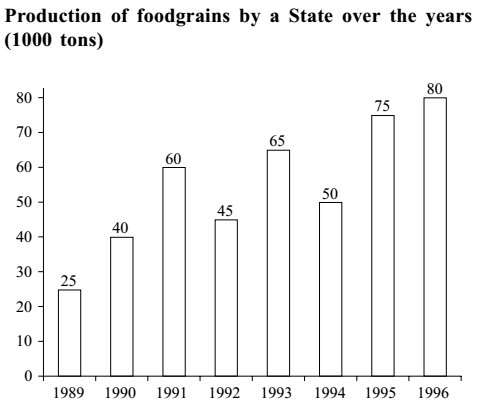## Introduction to Data Interpretation

#### Data Interpretation

Direction: Study the following graph carefully and answer the questions given below it.1. The average production of 1990 and 1991 was exactly equal to the average production of which of the following pairs of years?

1. As per given graph in question,
The average production of 1990 and 1991 = (40 + 60)/2 = 100/2 = 50
try to solve all given option one by one.

##### Correct Option: E

As per given graph in question,
The average production of 1990 and 1991 = (40 + 60)/2 = 100/2 = 50
The average production of 1991 = 60
Solve one by one option.
(A) The average production of 1991 and 1992 = (60 + 45)/2 = 105/2 = 52.5
(B) The average production of 1992 and 1994 = (45 + 50)/2 = 95/2 = 47.5
(C) The average production of 1993 and 1994 = (65 + 50)/2 = 115/2 = 57.5
(D) The average production of 1994 and 1995 = (50 + 75)/2 = 125/2 = 62.5

All the given option doesn't have average production of 50. So answer will be none of these.

1. What was the difference in the production of food-grains between 1991 and 1994?

1. Difference in the production of food-grains between 1991 and 1994 = Production of food-grains in 1991 - Production of food-grains in 1994

##### Correct Option: A

Difference in the production of food-grains between 1991 and 1994 = Production of food-grains in 1991 - Production of food-grains in 1994
Put the value by seeing the graph
Difference in the production of food-grains between 1991 and 1994 = ( 60 − 50 ) x 1000 = 10 x 1000 = 10,000

1. In which of the following years was the percentage increase in production from the previous year the maximum among the given years?

1. Check given option one by one.
Option (A),
Percentage increase in production in 1991 = {(Production in 1991 - Production in 1990)} x 100 % / Production in 1990
Percentage increase in production in 1991 = {( 60 - 40 )} x 100 % / 40
Percentage increase in production in 1991 = 20 x 100 % / 40
Percentage increase in production in 1991 = 100 % / 2 = 50 %

##### Correct Option: D

Check given option one by one.
Option (A),
Percentage increase in production in 1991 = {(Production in 1991 - Production in 1990)} x 100 % / Production in 1990
Percentage increase in production in 1991 = {( 60 - 40 )} x 100 % / 40
Percentage increase in production in 1991 = 20 x 100 % / 40
Percentage increase in production in 1991 = 100 % / 2 = 50 %
Option (B)
Percentage increase in production in 1993 = {( Production in 1993 - Production in 1992 )} x 100 % / Production in 1992
Percentage increase in production in 1993 = {( 65 - 45 )} x 100 % / 45
Percentage increase in production in 1993 = 20 x 100 % / 45
Percentage increase in production in 1993 = 4 x 100 % / 9 = 400/9 = 44.44 %
Option (C)
Percentage increase in production in 1995 = {( Production in 1995 - Production in 1994 )} x 100 % / Production in 1994
Percentage increase in production in 1995 = {( 75 - 50 )} x 100 % / 50
Percentage increase in production in 1995 = 25 x 100 % / 50
Percentage increase in production in 1995 = 100 % / 2 =50 %
Option (D)
Percentage increase in production in 1990 = {( Production in 1990 - Production in 1989 )} x 100 % / Production in 1989
Percentage increase in production in 1990 = {( 40 - 25)} x 100 % / 25
Percentage increase in production in 1990 = 15 x 100 % / 25
Percentage increase in production in 1990 = 15 x 4 % = 60 %

1. What was the percentage drop in the production of food-grains from 1991 to 1992?

1. Required percentage drop = Percentage decrease in production in 1991 to 1992 = {(Production in 1992 - Production in 1991)} x 100 % / Production in 1991

##### Correct Option: C

Required percentage drop = Percentage decrease in production in 1991 to 1992 = {( Production in 1992 - Production in 1991 )} x 100 % / Production in 1991

 = 60 - 45 X 100 = 25 % 60

1. In how many of the given years was the production of foodgrains more than average production of the given years?

1. Average production

 = 15 + 40 + 60 + 45 + 65 + 50 + 70 + 80 8

##### Correct Option: C

Average production

 = 15 + 40 + 60 + 45 + 65 + 50 + 70 + 80 8

 = 440 = 55 . 8

The average production of the year is 55 and as we are seeing in the graph that the year 1991, 1993, 1995, 1996 have more than the 55.